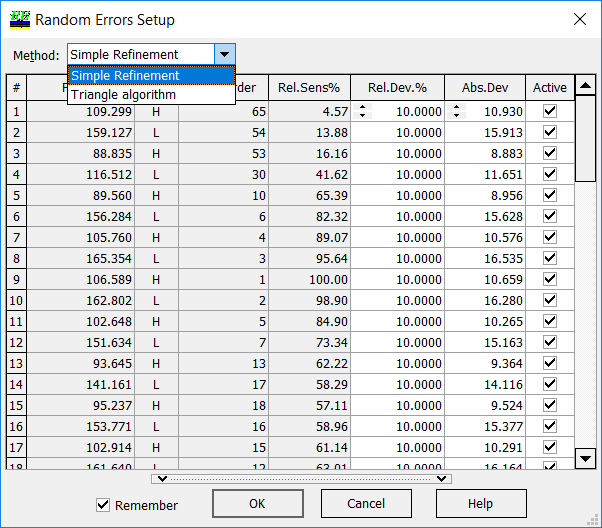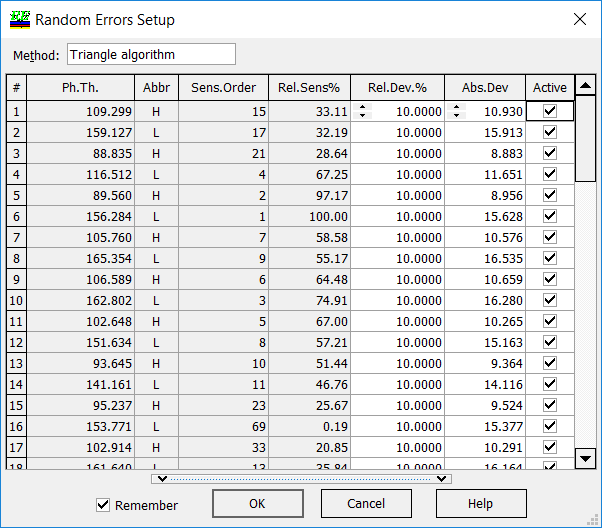Evolutionary Reverse Engineering Algorithm

 New evolutionary post-production characterization algorithm significantly improves the search for the best fit in the case of multi-scan data. The new option is available in OptiRE through:  Solve --> Random Errors Solve --> Quasi-Random Errors Solve --> Quasi-Random Inhomogeneities Solve --> Random Errors (scientific mode) Solve --> Quasi-Random Errors (scientific mode) In comparison with the previous versions, there is a choice between two algorithms in "Method" field: Simple Refinement and Triangular algorithm. In the case of "Simple Refinement", discrepancy function $$DF$$ is minimized with respect to all "Active" layer thicknesses: $DF^2=\frac{1}{m}\sum\limits_{k=1}^m\frac 1L\sum\limits_{j=1}^L\left[\frac{T(d_1,...,d_m;\lambda_j)-\hat{T}^{(k)}(\lambda_j)}{\Delta T_j}\right]^2,$ where $$m$$ is the number of design layers, $$\{\lambda_j\}, \; j=1,...,L$$ is the wavelength grid, $$\hat{T}^{(k)}(\lambda_j)$$ transmittance scan recorded after the deposition of $$k-$$th layer, $$\Delta T_j$$ are measurement tolerances.In the case of "Triangular algorithm", partial discrepancy functions $$DF(i)$$ are minimized with respect to all "Active" layer thicknesses among $$d_1,...,d_i$$: $DF^2(i)=\frac{1}{i}\sum\limits_{k=1}^i\frac 1L\sum\limits_{j=1}^L\left[\frac{T(d_1,...,d_i;\lambda_j)-\hat{T}^{(k)}(\lambda_j)}{\Delta T_j}\right]^2,$ where $$m$$ is the number of design layers, $$\{\lambda_j\}, \; j=1,...,L$$ is the wavelength grid, $$\hat{T}^{(k)}(\lambda_j)$$ transmittance scan recorded after the deposition of $$k-$$th layer, $$\Delta T_j$$ are measurement tolerances. Important: At each $$i-$$step, starting approximations of actual layer thicknesses are taken from the result of the $$(i-1)-$$step. This reduces the instability in determination of actual layer thicknesses significantly.

Easy to startOptiLayer provides user-friendly interface and a variety of examples allowing even a beginner to effectively start to design and characterize optical coatings.        Read more...

Docs / SupportComprehensive manual in PDF format and e-mail support help you at each step of your work with OptiLayer.If you are already an experienced user, OptiLayer gives your almost unlimited opportunities in solving all problems arising in design-production chain. Visit our publications page and challenge page.